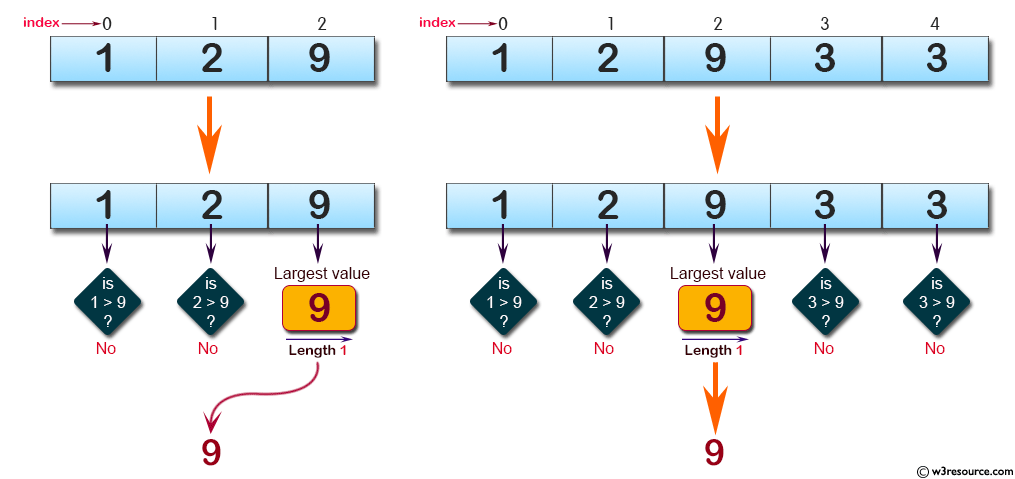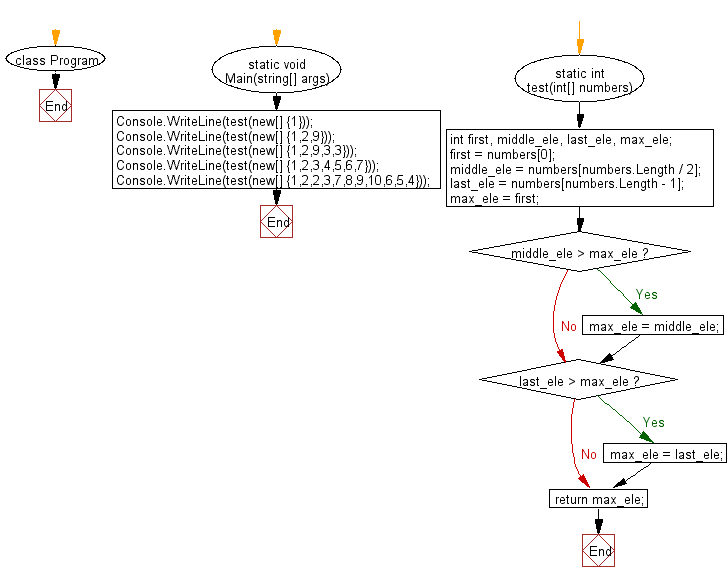﻿ C# - Largest value of the first, last, middle of an array# C# Sharp Basic Algorithm Exercises: Find the largest value from first, last, and middle elements of a given array of integers of odd length (at least 1)

## C# Sharp Basic Algorithm: Exercise-107 with Solution

Write a C# Sharp program to find the largest value from first, last, and middle elements of a given array of integers of odd length (at least 1).

Pictorial Presentation:Sample Solution:-

C# Sharp Code:

``````using System;
namespace exercises
{
class Program
{
static void Main(string[] args)
{
Console.WriteLine(test(new[] {1}));
Console.WriteLine(test(new[] {1,2,9}));
Console.WriteLine(test(new[] {1,2,9,3,3}));
Console.WriteLine(test(new[] {1,2,3,4,5,6,7}));
Console.WriteLine(test(new[] {1,2,2,3,7,8,9,10,6,5,4}));

}
static int test(int[] numbers)
{
int first, middle_ele, last_ele, max_ele;

first = numbers;
middle_ele = numbers[numbers.Length / 2];
last_ele = numbers[numbers.Length - 1];
max_ele = first;

if (middle_ele > max_ele)
{
max_ele = middle_ele;
}

if (last_ele > max_ele)
{
max_ele = last_ele;
}

return max_ele;
}
}
}
```
```

Sample Output:

```1
9
9
7
8```

Flowchart:C# Sharp Code Editor:

Improve this sample solution and post your code through Disqus

What is the difficulty level of this exercise?

Test your Programming skills with w3resource's quiz.

﻿